1. Price Delimiter

The price Delimiter provided in the cost field has two options one is Comma and Decimal Point.2. Price Round

Price Round is also available in the cost field. You can choose any that is suitable for you.3. Cost Condition

How to use Cost Conditions according to the Examples below:

The first tab is the statement and the second tab is the condition.`This condition will not update when the price in your store is 0 in the feed file``If the price is 11, then the price is + 1`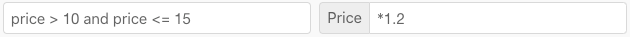`If the price is more than 10 and equals or below 15, then the price is multiplied by 1.2 or 20%``All the prices will be multiplied by 1.5 or 50%``If the price is more than column 1, then add column 1 and column 2 to the price`
`Use col#_f when the comparison values are numeric`
`col expression is not available for the XML file format`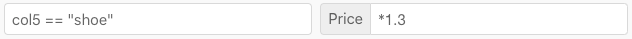`If column 5 has value shoe, then multiply by 1.3. This can be used for any column and is suitable if you are planning to add markup based on collections``If the price is less than 1, then it will use the current price in store (Shopify only)`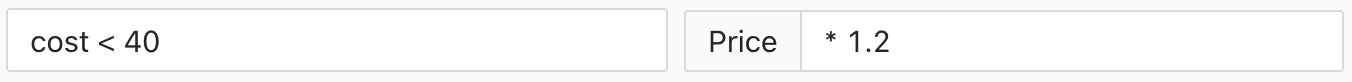`If cost in the store is less than 40, then multiply the price by 1.2. The cost value will be taken from the store. (Shopify only)`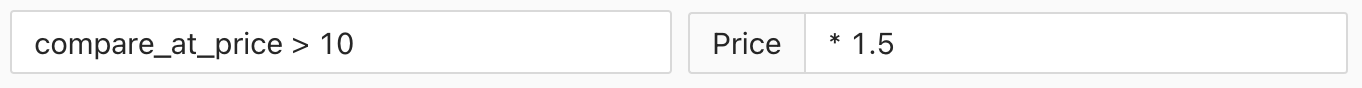`If compare at price in the store is more than 10, then multiply the price by 1.5. The compare at price value will be taken from the store. (Shopify only)`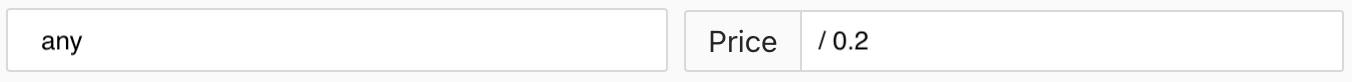`All the prices will be divided by 0.2`

4. Don't update Cost when...

For the cost condition, there is another way that can filter the cost only according to the vendor, product type, and product tags. You can choose any one of the methods to filter the price.1. The Cost Conditions take effect according to the arrangement. The first conditions have the highest priority.

2. Stock Sync now supports Column Value.

3. Valid price range keywords are:

• price
• any
• current_price
• cost

4. The symbol that is used for the statement:

• == equal to
• <= less than or equal to
• => more than or equal to
• and
• or